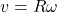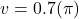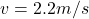## if 130N centripetal force is needed to keep a 0.45kg ball that is attached to a string that is 0.7m long to complete 5 full rotations every

Question

if 130N centripetal force is needed to keep a 0.45kg ball that is attached to a string that is 0.7m long to complete 5 full rotations every 10 seconds. what is the centripetal acceleration and velocity felt by the ball?

in progress 0
1 month 2021-08-14T06:24:28+00:00 1 Answers 0 views 0

centripetal acceleration of the ball is 6.9 m/s/s

tangential speed of the ball is 2.2 m/s

Explanation:

As we know that ball complete 5 rotations in 10 seconds

so frequency of rotation of ball is given as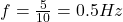now we know that angular frequency is given as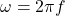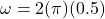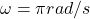Now centripetal acceleration is given as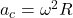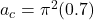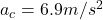now the velocity of the ball at this angular frequency is given as